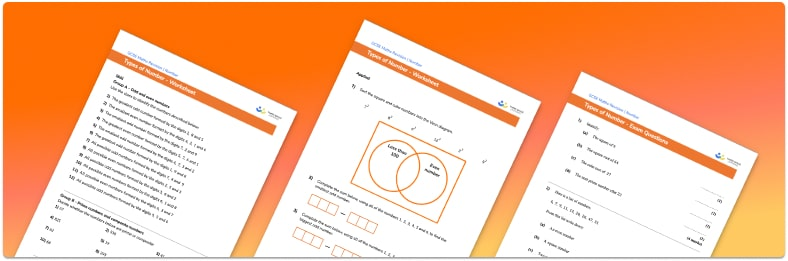# Types of numbers Worksheet• Section 1 of the types of numbers worksheet contains 20+ skills-based types of numbers questions, in 3 groups to support differentiation
• Section 2 contains 3 applied types of numbers questions with a mix of worded problems and deeper problem solving questions
• Section 3 contains 3 foundation and higher level GCSE exam style types of numbers questions
• Answers and a mark scheme for all types of numbers questions are provided
• Questions follow variation theory with plenty of opportunities for students to work independently at their own level
• All questions created by fully qualified expert secondary maths teachers
• Suitable for GCSE maths revision for AQA, OCR and Edexcel exam boards

• This field is for validation purposes and should be left unchanged.

You can unsubscribe at any time (each email we send will contain an easy way to unsubscribe). To find out more about how we use your data, see our privacy policy.

### Types of numbers at a glance

There are lots of different types of numbers on the number line. Rational numbers are numbers that can be written as a fraction where the numerator and denominator are integers, whereas irrational numbers cannot be written in this way.

Integers are whole numbers that include positive and negative numbers, and natural numbers (sometimes called counting numbers) are positive integers. Fractions, decimals and percentages are different ways of representing a proportion of the same amount.

Even numbers can be divided by 2 with a remainder of 0 whereas odd numbers cannot. Prime numbers only have two factors, themselves and 1, whereas composite numbers have more than two factors.

Multiples are the result of multiplying a number by an integer and factors are numbers that will divide into an integer with no remainder.

Looking forward, students can then progress to additional number worksheets, for example a Percentage worksheet or a Rounding worksheet.For more teaching and learning support on Number our GCSE maths lessons provide step by step support for all GCSE maths concepts.

## Do you have KS4 students who need more focused attention to succeed at GCSE?There will be students in your class who require individual attention to help them succeed in their maths GCSEs. In a class of 30, it’s not always easy to provide.

Help your students feel confident with exam-style questions and the strategies they’ll need to answer them correctly with our dedicated GCSE maths revision programme.

Lessons are selected to provide support where each student needs it most, and specially-trained GCSE maths tutors adapt the pitch and pace of each lesson. This ensures a personalised revision programme that raises grades and boosts confidence.

Find out more